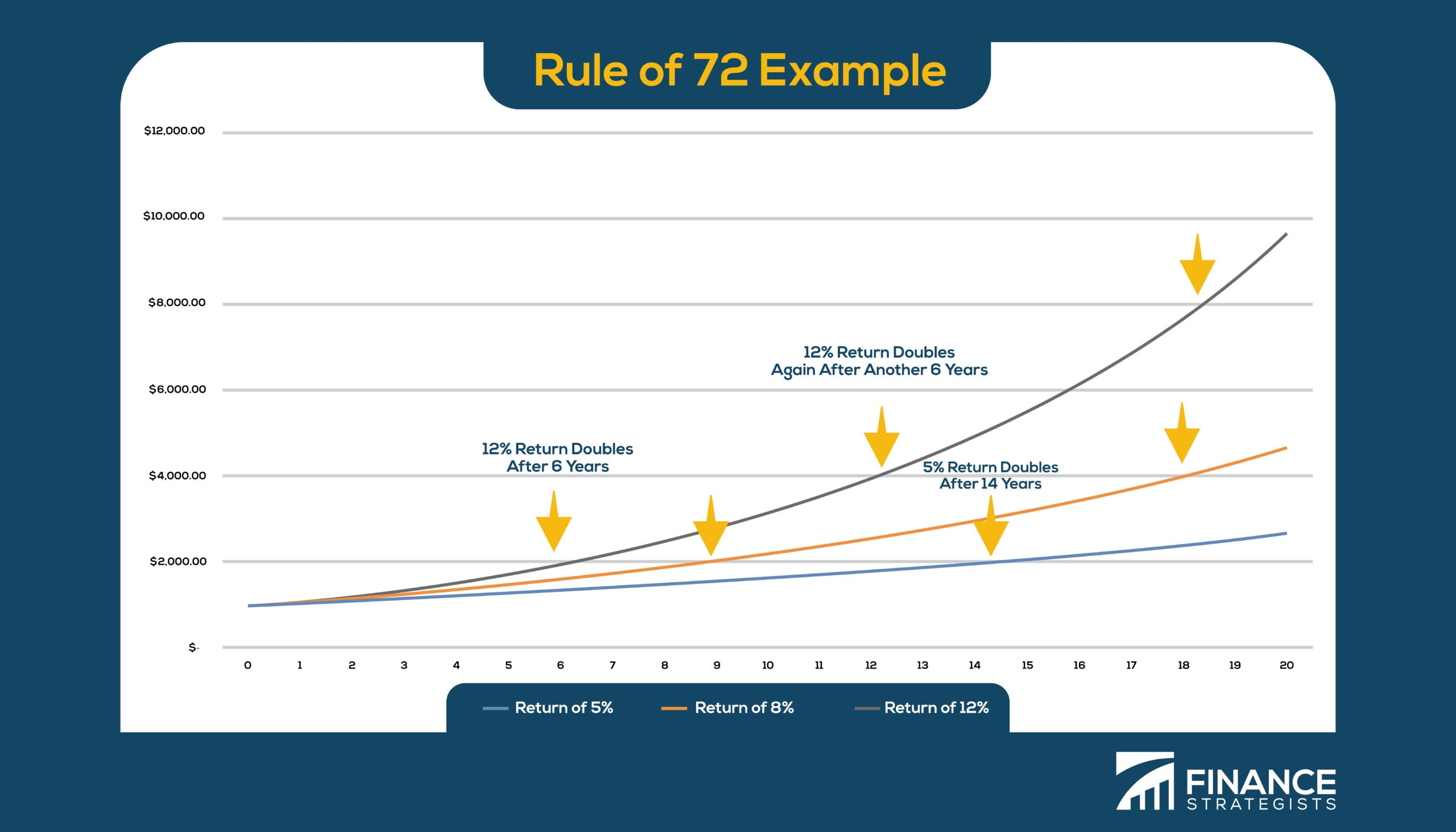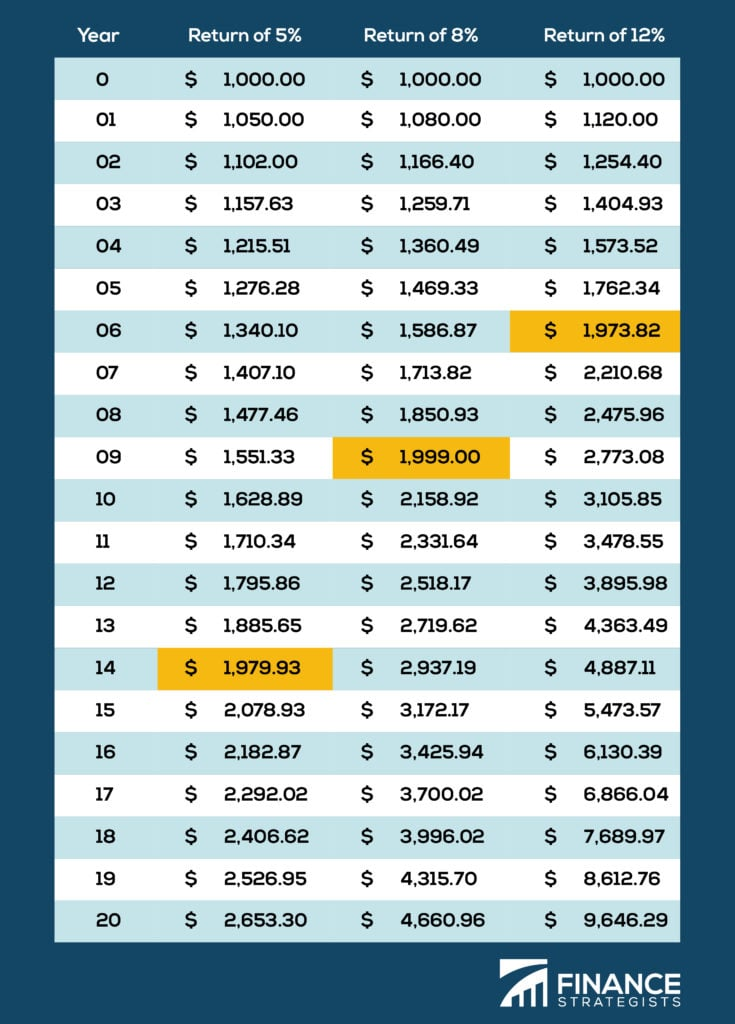# What Is the Rule of 72?### Reviewed by Subject Matter Experts

Updated on June 08, 2023

## What Is the Rule of 72?

The Rule of 72 is a finance shortcut to quickly estimate how long an investment will take to double.

The Rule of 72 definitions can be described as simple as dividing 72 by the rate of return an investment earns.

This is the number of periods it will take the investment to double in value.

## What’s an Example of the Rule of 72?

For example, according to the Rule of 72 formula, an investment of \$100 that earns 7% annually (compounded) will take 10.3 years to be worth \$200 because 72/7 = 10.3.

The Rule of 72 can also be used to calculate the cost of debt.

A credit card balance of \$1,000 at a 25% APR will be a balance of \$2,000 in 2.88 years because 72/25 = 2.88.

The Rule of 72 can be used in the opposite direction to estimate the rate if the amount of time is known.

For example, if you wanted to double \$1,000 in 3 years, you would need to earn an interest rate of 24% because 72/3 =24.The graph shows the time it takes different investments to double. Tip: Look at where the arrows are pointing (\$2,000, \$4,000, \$8,000) to show doubling in return and the year at which it occurs according to the interest rate.

## When Would You Need to Use the Rule of 72?

The "rule" is really a "rule of thumb" or approximation used for quick mental math.

In our first example, the Rule of 72 told us the investment would double in 10.3 years.

In actuality, the investment would only take 10.24 years to double.

Higher interest rates and longer time frames cause the Rule of 72 to become less accurate.

## Rule of 69.3 and Rule of 70

The Rule of 69.3 and Rule of 70 work similarly and are used for different scenarios.

If the investment is compounding continuously rather than compounding annually, the Rule of 69.3 offers a more accurate estimation.

To determine the time it takes for money's buying power to halve due to inflation, financiers divide 70 by the inflation rate, which is the Rule of 70.

## Rule of 72 ChartThe chart highlights how many years it takes an investment to double using different interest rates.

## Rule of 72 FAQs